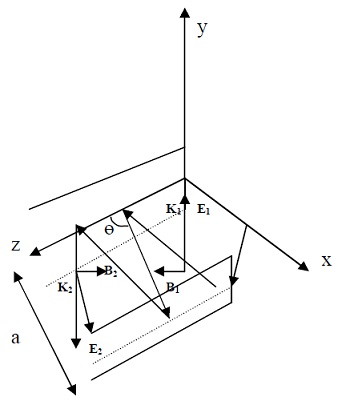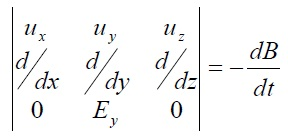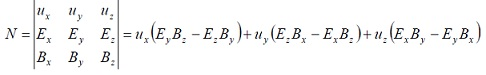#### Waveguides, Physics tutorial

Waveguides:

Waveguides are utilized for same objectives as transmission lines i.e. to transmit electromagnetic energy. Though, waveguides are utilized at microwave frequencies and they are not as lossy as transmission lines that are utilized at lower frequencies. There are two kinds of waveguides, the metal tubes of any cross section and dielectric rods. Wave travels on inside of the first type and on outside of the other.

Rectangular waveguide:

Rectangular waveguide is hollow infinite rectangular pipe of highly conducting material. Rectangular waveguide is composed of two pairs of planes. For sake of simplicity a pair of planes will be considered first.

Transmission of waves in a pair of parallel conducting:

Planes:

Let separation between pair of planes be distance, d, assuming planes are perfectly conducting and of infinite length, features of electric and magnetic fields which can travel down between planes have to be determined. It is essential for fields to obey Maxwell's equations in free space in between plates. The following are boundary conditions on planes.

(i) Parallel or tangential component of electric field and perpendicular or normal component of magnetic field should be zero at every point on planes. This is because there is no electromagnetic field inside perfect conductors.

(ii) Perpendicular or normal component of electric field and parallel or tangential component of magnetic field need not equal zero. This is due to there can be charges on surfaces of plane, and surface currents in them.

Obeying Maxwell's equations implies satisfaction of electric field wave equations and magnetic field wave equations i.e.

2E = ε0μ0d2E/dt2

2B = ε0μ0d2B/dt2

Linearly polarized plane wave whose electric and magnetic fields, respectively are

E = exE0exp[jw(t-kz/w)]

B = -eyE0/cexp[jw(t - kz/w)]

It is not only solution to equations but also satisfies boundary conditions (i) & (ii) stated above.

Linearly polarized wave whose electric and magnetic fields are expressed above is transverse electric and magnetic (TEM) wave. This is due to both fields are not only perpendicular to each other but are also transverse to direction of propagation such that three are mutually perpendicular i.e. orthogonal. Although TEM waves can travel in pair of planes just as they could in parallel wire and coaxial cable transmission lines, they can't, though, be propagated in wave guide which is a hollow conductor. This is because perfectly conducting walls of waveguide will short circuit the electric field, electric potential not being able to exist across perfect conductors.Superposition of incident and reflected waves whose electric fields and magnetic fields are represented by E1 and B1, and E2 and B2 respectively in Figure is also solution to equations.

If E1 oscillates along y - direction, its expression becomes

E1 = uyE0exp[jw(t - k1r/w)]

=uyE0exp[jw(t - r/w)]

Where v1 = -uxsinΘ/V + uzsinΘ/V

Such that 1/V1.r = -xsinΘ/v + zcosΘ/v

And equation becomes,

E1 = uyE0exp[jw(t - zcosΘ/v + xsinΘ/v)]

On reflection wave has velocity

1/V2 = uxsinΘ/v + uzcosΘ/v

Electric field, E2, of reflected wave is given by equation

E2 =-uyE0exp[jw(wt - zcosΘ/v - xsinΘ/v)]

Superposing E1 and E2 to obtain resultant field E provides

E = uy2jsin((wx/v) sinΘ)exp[jw(t - zcosΘ/v)]

Planes are placed at x = 0 and x = a as by our boundary conditions E = 0 at walls i.e. first at x = 0; substituting x = 0 in equation, E automatically becomes zero showing that boundary condition is satisfied. If Θ is chosen such that sin(wx/v sinΘ) = 0 then E = 0 at x = a. This satisfies boundary conditions on second plane. Condition that

sin((wx/v)sinΘ) = 0

Implies that wx/vsinΘ = nπ or sinΘ = nπv/wx

Putting sinΘ≤1 and x = a we have, nvπ/wa≤1

Suppose vπ/wa ≥ 1 when n =1, λ/2a≥1 or f≤c/2a

f is cut- off frequency when n = 1. For n>1, fn = nc/2a. For instance when pair of planes is separated by 2cm, from equation i.e. sinΘ = nvπ/wx

CosΘ = [1 - (nvπ/wx)2]1/2

Substituting sin θ and cos θ into equation provides

E = uy2jE0sin(nπx/a)exp[jw(t-z/Vg)]

Where 1/Vg = (1/V2 - n2π2/w2b2)1/2

Using fact that 1/V = k/w

1/Vg = kg/w and kg = (k2 - n2π2/a)1/2

Electric field components for TE waves are

Ey = 2jE0sin(nπx/a)exp[jw(t-z/vg)]

Ex = 0 and Ez = 0

By differential form of Faraday's law i.e. ∇xE = dB/dt we can get components of magnetic fields by usingWe have

ux(-dEy/dz) + uz(dEy/dx) = -dB/dt or

dB/dt = jw/vg.2jE0sin(nπx/a)exp[jw(t-z/vg)]ux - (nπ/a).2jE0cos(nπx/a)exp[jw(t-z/vg)]uz

Integrating provides

Bx = (2j/vg)E0sin(nπx/a)exp[jw(t - z/vg)]

By = 0

Bz = -(2nπ/wa)E0cos(nπx/a)exp[jw(t-z/vg)]

= - 2(1/v2 - 1/v2g)1/2E0cos(nπx/a)exp[jw(t-z/vg)] where 1/v2 - 1/v2g = n2π2/w2a2

We can also add two waves having their magnetic field in y-direction only such that

B1 = eyB0exp[jw(t - z/vg)] and B2 = -eyB0exp[jw(t-z/vg)]

Superposing two fields gives the set of equations in which resultant B has only y-component, By; Bx and Bz being equal to zero. Electric field, E will then have component in z-direction. Such a wave is known as transverse magnetic (TM) wave.

Waveguide:

Two pairs of parallel conducting planes form a rectangular waveguide. There two types of waveguides- the metal tubes of any cross section and the dielectric rods. Wave travels on the inside of the first type and on the outside of the other. The second type is outside the scope of this course. Also, we limit our discussion to rectangular waveguides.

Adding another pair of perfectly conducting parallel planes to the pair already treated such that these new planes are located at y = 0 and y = b, we obtain the waveguide shown in Figure 2. The waveguide is a hollow rectangular metal pipe. Only the TE and TM waves can propagate in the waveguide. By the presence of the new pair of parallel plates separated along y - axis, the electric field is expected to also vary in the y - direction in addition to its variation in the x - direction such that,

E ∝ Kf(y)sin(nπx/a)exp[jw(t-z/v)]

Or E = Kf(y)sin(nπx/a)exp[t - z/v]

For wave to be able to propagate in waveguide, equation should satisfy equation electric field wave equation and Maxwell's equations. Taking 1st Maxwell's equation i.e. ∇.E = 0 or

dEx/dx + dEy/dy + dEz/dz = 0

Ez = 0 as wave can't have component in direction of propagation. Consequently dEz/dz = 0 therefore equation becomes

dEx/dx + dEy/dy = 0 or dEx/dx = -dEy/dy

dEx/dx = -K(df(y)/dy)sin(mπx/a)exp[jw(t-z/v)]

By integration Ex becomes

Ex = (ca/mπ)(df(y)/dy)cos(mπx/a)exp[jw(t-z/v)]

We can find out f (y) by making Ex satisfy boundary conditions that it be zero at walls y = 0 and y = b and satisfy wave equation and ensuring that wave equation is satisfied by Ex.

For Ex = 0 at y = 0, (a/mπ)(df(y)/dy)=sinky = sinnπ or df(y)/dy = (mπ/a)sinky = (mπ/a)sinmπ

Putting y = b, nπ = kb or k = nπ/b, and, df(y)/dy = (mπ/a)sin(nπy/b)

Integrating df(y)/dy we have

f(y) = -(mπ/a)cos(nπy/b)

For pair of parallel plates we had,

kg2 = k2 - (n2π2/b2 + (m2π2/a2))

or k2 - kg2 = (n2π2/b2 + (m2π2/a2)) which is waveguide equation.

Guide wave number, kg, from which cut-off wave length is determined for particular mode is obtained from dimensions (width and length) of wave guide and free space wave number. We can write equation as

k2c = k2 - kg2 where

kc2 = m2π2/a2 + n2π2/b2 or 4π2c2 = m2π2/a2 + n2π2/b2

Therefore equation can be written as:

2c2 = 4π22 - 4π2g2 or 1/λc2 = 1/λ2 - 1/λg2

Substituting for f(y) in equations  we obtained components of electric field as shown below.

Ex = (knπ/b)sin(nπy/b)cos(mπx/a)exp[jw(t-z/v)]

Ex = (kmπ/b)cos(nπy/b)sin(mπx/a)exp[jw(t-z/v)]

Ez = 0

That Ez = 0 is really the reason the wave is referred to as TE as it is transverse to direction of propagation i.e. z-direction having no component in that direction. Expressions for magnetic fields are obtained from differential form of Faraday's law i.e. ∇xE = -dB/dt as follows:

Bx = -(kgKmπ/wa)cos(nπy/b)sin(mπx/a)exp[jw(t-z/v)]

Bx = -(kgKnπ/wb)sin(nπy/b)cos(mπx/a)exp[jw(t-z/v)]

Bz = jw(1/v2 - 1/v2g)cos(nπy/b)cos(mπx/a)exp[jw(t-z/v)]

Direction of propagation is z-direction. Therefore energy of wave is in z-direction. Rate of energy flow across unit area is referred to as poynting vector, N. it can be illustrated that N is vector product of electric field and magnetic field of wave i.e.

N = ExB

Expanding equation (4), we have,Thus,

Nx = EyBz - EzBy = EyBz since Ez = 0.

Ny = EzBx - ExBz = -ExBz since Ez = 0, and

Nz = ExBy - EyBx

As direction of energy is z-direction as mentioned above, Nx = Ny = 0 i.e. EyBz = ExBz = 0 implying that Ey and Bz are out of phase and Ex and Bz are out of phase. Energy produced by the products only flows in and out of the planes. Cut-off frequency of TE waves is obtained from equation and fc = c/λc to give

fc = c/2√(m/a)2 + (n/b)2

Only waves of frequency greater than fc can be propagated in guide of given a×b. Therefore wave guide behaves like high-pass filter. In other words only modes whose cut-off frequencies are lower than radiation frequency can be propagated.

Transverse Magnetic (TM) waves in which there is no magnetic field in direction of propagation are also propagated in guide. They should, though, satisfy boundary conditions of guide and wave equation. For these waves electric field has component in direction of propagation. TM modes would have cut-off frequencies at same values as TE waves given by equation.

We should though note that unlike TE there cannot be TMon or TMmo. This is due to field lines of magnetic field in waveguide is two-dimensional there being no magnetic charges or mono poles and as such magnetic field has to differ with both coordinates other than in direction of propagation. Therefore lowest TM modes which can exist is TM11 mode. Electric field, on other side is one dimensional in guide. As such its lines of force go from one plane to another. Therefore lowest TE mode is either TE01 or TE10 depending on which of width, a, or height, b, is longer. For example cut-off frequencies for TE01 i.e. f01 = c/2√(1/b)2 = c/2b

While that of TE01 i.e. f01 = c/2√(1/a)2 = c/2a

Lowest TE mode is fundamental and significant mode in rectangular waveguide. Take for instance waveguide of dimensions a = 3 cm and b= 1.5cm in which wave whose wavelength in free space is 5cm corresponding to the frequency of 6 × 109Hz i.e. 6GHz, cut-off frequencies of given modes are determined and compared. It is only f10 that is lower than frequency of propagated wave. As such it is only f10 that is permitted in guide. Note that f10 is fundamental and most significant mode. Velocity with which wave crests and/or troughs travel through medium is called as phase velocity, vp. From fact that velocity is quotient of angular frequency and wave number i.e. v = w/k, phase velocity in a wave guide is vp = w/kg (kg being guide wave number). kg can be determined from combination of equations from which we can get vp. In example above vp = 5.5×108ms-1 and vp > c (velocity of light)

Group velocity, vg is velocity of group of waves whereas phase velocity is velocity of individual waves within group. Waves within group are observed to travel faster than group itself, therefore vp > vg. It is, though, group velocity which is usually estimated as wave velocities are estimated by arrival of disturbances and it is velocity by which energy of wave is propagated. vg < c and as such there is no contradiction of Einstein's. The product of phase velocity and group velocity equals c2. Therefore vg = c/vp = 1.64ms-1.

The poynting vector of guide in direction of propagation i.e. Nz for TE10 mode = EyHx = (kg/wμ0)E2ysin2(πx/a) by combining expressions of Ey and Bx above and noting that B = Hx0. For TE10 mode, m = 1 while n = 0. Substituting for m and n in expressions of Ex and By above shows Ex = By = 0 such that Nz = EyBx implying that first term of left hand side of equation is zero. Average power of operation of the guide for TE10 mode is therefore provided by (1/2)(kg/μ0w)E102(ab/2), kg, a b and w are obtainable from wavelength and guide dimension known; electric field, E, would be given and average power can then be obtained. Integrating expression over area of the guide, we get

(1/2)(kg/wμ0)Eoy2 sin2(nπ/a)

Tutorsglobe: A way to secure high grade in your curriculum (Online Tutoring)